Next: Method of Averaging Up: Charged Particle Motion Previous: Introduction

# Motion in Uniform Fields

Let us, first of all, consider the motion of charged particles in spatially and temporally uniform electromagnetic fields. The equation of motion of an individual particle takes the form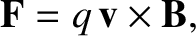(36)

The component of this equation parallel to the magnetic field,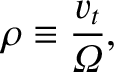(37)

predicts uniform acceleration along magnetic field-lines. Consequently, plasmas near equilibrium generally have either small or vanishing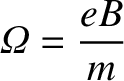.

As can easily be verified by substitution, the perpendicular component of Eq. (36) yields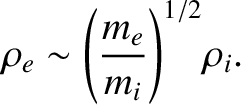(38)

whereis the gyrofrequency,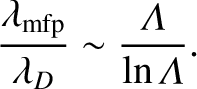is the gyroradius,and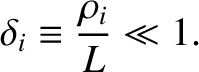are unit vectors such that (,,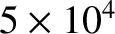) form a right-handed, mutually orthogonal set, and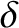is the initial gyrophase of the particle. The motion consists of gyration around the magnetic field at frequency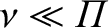, superimposed on a steady drift at velocity(39)

This drift, which is termed the E-cross-B drift by plasma physicists, is identical for all plasma species, and can be eliminated entirely by transforming to a new inertial frame in which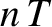. This frame, which moves with velocity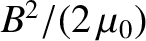with respect to the old frame, can properly be regarded as the rest frame of the plasma.

We complete the solution by integrating the velocity to find the particle position:(40)

where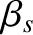(41)

and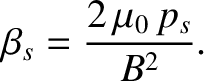(42)

Here,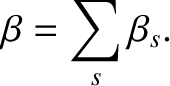. Of course, the trajectory of the particle describes a spiral. The gyrocentre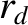of this spiral, termed the guiding centre by plasma physicists, drifts across the magnetic field with velocity, and also accelerates along the field at a rate determined by the parallel electric field.

The concept of a guiding centre gives us a clue as to how to proceed. Perhaps, when analyzing charged particle motion in non-uniform electromagnetic fields, we can somehow neglect the rapid, and relatively uninteresting, gyromotion, and focus, instead, on the far slower motion of the guiding centre? Clearly, what we need to do in order to achieve this goal is to somehow average the equation of motion over gyrophase, so as to obtain a reduced equation of motion for the guiding centre.Next: Method of Averaging Up: Charged Particle Motion Previous: Introduction
Richard Fitzpatrick 2011-03-31